How Does Universal Gravitation Arise

How Does Universal Gravitation Arise

Li huawang

Abstract

Great principles are extremely simple, and looking for the essence of physics requires us to trace the roots. Binary can be arranged to combine our information world, then would the three elementary particles  combine to form the periodic table of elements? Without giving them additional attributes (such as: electricity, magnetism, gravity, etc.), would they further arranged this world? This paper proved the existence of a new particle through three physical experiments. The mass of this new particle is only 10-20 of the electron, and it is named Yizi. Through the discovery of Yizi, the reason of universal gravitation is revealed and the essence of force is expounded.

Experiment 1: The gravitational force between two objects is not only related to their masses but also to their ambient temperature. The force between two objects is mutually exclusive when their temperature is lower than ambient.

Experiment 2: Change the temperature of the big metal ball on the universal gravitation torsion scale,,keeping the temperature of the small metal ball unchanged, and observe the force of the big metal ball on the small metal ball.

Experiment 3: Under constant temperature and vacuum conditions, the temperature of an object is related to its mass. The larger its mass, the higher its temperature.

Keywords: the essence of force, universal gravitation, elementary particles.

Introduction

Physics is the cornerstone of human civilization. In recent years, the development of physics is in a state of stagnation. Faced with many new physical experimental phenomena, physicists are hard to justify themselves. Where did it all go wrong? To find out what went wrong, we must start with the most elementary particles that make up matter, determine the correct properties of the elementary particles, and then we will find the roots of matter.

There are a lot of colorful interstellar clouds  suspended in the vast universe, which seem to be set off by a kind of gas, just like the clouds set off by the air. Will there be such a kind of gas? In an electron cyclotron , would a speeding electron be slowed down by the resistance of a gas in the vacuum? Will the propagation of light be transmitted through a gas like air-borne sound? Will gravitation be from the buoyancy of a gas, just like the buoyancy of air to an object? The atmospheric pressure generated by the air can squeeze two objects together, but people don't feel the presence of atmospheric pressure. Then, will the nucleus be squeezed together by a gas with a very high pressure? The electrons do Brownian motion around the nucleus , will it be caused by this gas pressure? Is the cosmic background radiation 2.735K  the microwave generated by this gas? Under normal temperature and pressure, the average mass of air molecules is 29g/mol, and the average kinetic energy of an air molecule is 6.02×10-21J, then will the Planck constant h=6.626×10-34  be the average kinetic energy of this gas particle in vacuum? At normal temperature and pressure, the average velocity of air molecules is 500m/s, the speed of sound propagation is 340m/s, and the speed of light propagation is 2.99792458×108 m/s .Will the average rate of this gas particle in vacuum be v=c=4.24×108 m/s or not?

There are indications that our space is filled with a gas like air, and the particles that make up this gas we name it Yizi. The difference between Yizi and Ether is that Yizi has mass and kinetic energy, and its average kinetic energy is Planck's constant. The movement of Yizi follows the conservation of energy and the law of conservation of momentum. Three physical experiments will be introduced here to prove the existence of Yizi.

1. The essence of force

Force is a macroscopic concept. The amount of change in the momentum of a group of particles per unit time is the amount of force generated by this group of particles. Let's look at an experiment. Making small balls as particle models, hold the cup with balls 5cm above the scale, pour 1 ball on the scale pan, the pointer of thescale will swing once. And then at the same height pour 100 or more balls on the scale pan continuously and quickly, as shown in Fig. 1, the pointer will swing around a position. This shows that a large number of balls hitting the scale pan produce a constant and uniform pressure on the scale. The more balls that collide during a certain period of time, theFig.1 The small balls slip onto the electronic scale.

greater the pressure on the scale pan. If the balls are poured from the higher position above the scale pan, it can be observed that the pointer of the scale indicates a greater pressure. This indicates that the greater the momentum of the balls are, the greater the pressure on the scale pan will be. The above is a description of the concept of force in junior high school physics textbook. In fact, this is the essence of force. However, when physicists face universal gravitation, electromagnetic force, weak interaction force, and strong interaction force, the essential concept of force is completely forgotten. The gravitation, electromagnetic force, weak interaction force and strong interaction force are all caused by the change of momentum due to particle collision. This is also the essence of force (the grand unified theory) .

2. The cause of gravitation

The universe is full of Yizi gas, and all the planets are suspended in it.The volatile Yizi gas rippled in space, forming the cosmic background temperature of 2.736K. However, the center temperature of each planet is very high, far higher than the background temperature of the universe, which makes the energy of Yizi gas near the planet especially high.The farther away from the planet, the lower the energy of Yizi gas.Where the energy of Yizi gas is high, the density Yizi gas is low, that is to say, the number of Yizi per unit volume is few, as shown in Fig.2: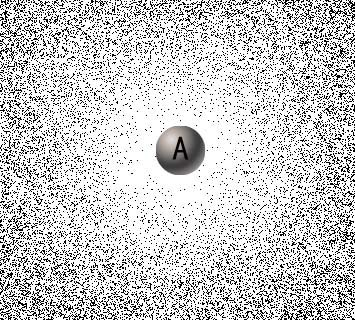FIG. 2 Distribution of Yizi gas around planet A

Where the energy of Yizi gas is low, its density is high and the number of Yizi gas per unit volume is large. The density of Yizi gas increases with the elevation, and it creates a top-down buoyancy. The amount of buoyancy an object receives depends only on the volume of the elementary particles that make up the object to displace the Yizi gas. If the quality of basic particle density are the same, then the buoyancy produced by the Yizi gas is related to the mass of the elementary particles that make up the object. This is why both the feather and the iron ball fell at the same time in the free fall experiment.

On earth, the gravitational force of one object on another is not always positive, but sometimes negative, meaning that one object repels another.When the temperature of an object B is lower than its ambient temperature, the distribution of the promoters around the object B is shown in figure 3: the kinetic energy of the promoters around the object B is small and the density is high, and then the object B has a repulsive effect on the object at the ambient temperature.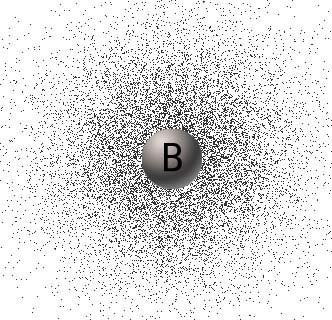Fig.3 The distribution of Yizi gas around object B when the temperature of object B is lower than the ambient temperature

You may ask if the earth's gravity decreases in a freezing winter. The earth's center temperature is very high, the radius of the earth is 6400 kilometers, and the sun can only change the earth's surface temperature about 10 meters deep.10 meters below the temperature is generated by the earth itself, the temperature is relatively constant, three feet of freezing is not enough to change the gravity of the earth.

Gravity can only exert a force within a certain distance. When an object is too far away from a planet, the space where it is located will have the same density of Yizi gas as the cosmic background temperature, and the force on the object will become zero.

Someone asked, if the temperature had such an effect on gravity, the rocket would have been so hot that it would have been impossible to control it. Right? When two objects attract each other, each changes the density of the Yizi gas around it, and  creates a gravitational field, the corresponding constant acceleration of gravity, such as the surface of the earth's gravitational acceleration g=9.8m/second2,and the gravitational acceleration constant produced by the rocket probably be only 10-9-10-8m/second2, negligible, that is to say, the contribution of earth and rocket to the magnitude of gravitation is not the same. The earth generates an active gravitational field.

Although the density of Yizi gas around the stars increases with the altitude, however in unit time, in any given radial direction of the planet, Yizi passes through a unit area. The number of Yizi diffuses from the inside of the unit area to the outside is equal to the number from the outside to the inside, both are n. Let Yizi’s outer-to-inner diffusion velocity be V1, V2, V3, ... Vn, the inner-to-outer diffusion velocity be V1', V2', V3', ... Vn', the average velocity of Yizi outer-to-inner diffusion be, and the average velocity of Yizi inner-to-outer diffusion be.Then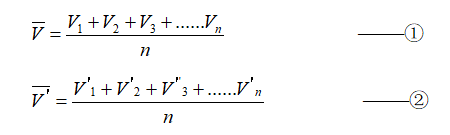LetV be the velocity variance of Yizi outer-to-inner diffusion, andV' be the velocity variance of Yizi inner-to-outer diffusion.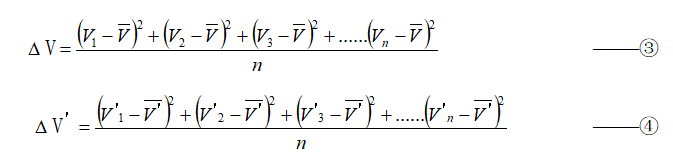The irregularity of a star decreases as the radius increases.The closer to the star, the greater the unevenness of the motion rate of Yizi is.So the unevenness of the motion rate that diffuses from the inside to outside is greater than that from the outside to inside, ie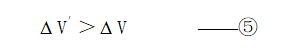Expand Equation 3 and Equation 4: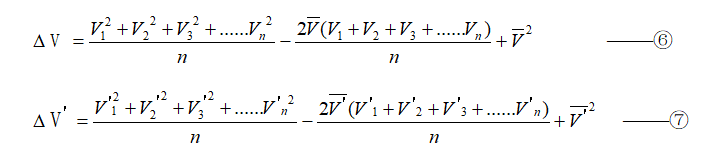Substituting Equation 1 and 2 into Equation 6 and 7 respectively: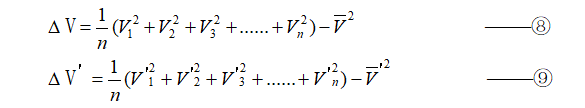Substituting Equation 8 and 9 into Equation 5 respectively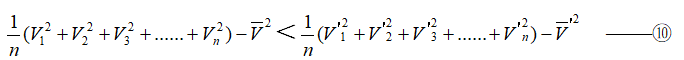According to the law of conservation of energy,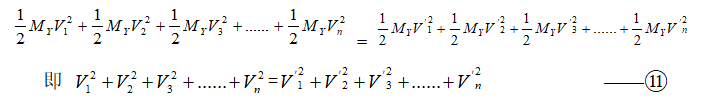Substituting Equation 11 into 10 gives: ie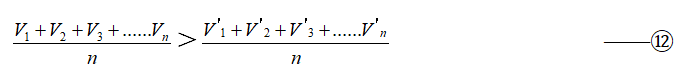multiplying both side of Equation 12 with ，we obtain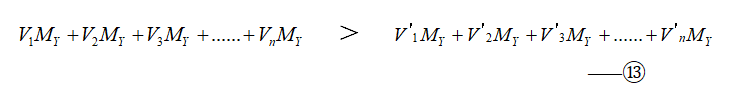Since force is a change in momentum per unit time, the force exerted by Yizi on this unit area: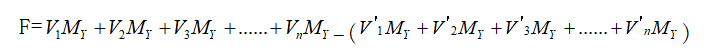3.  The Light and Heat of the Sun is not Generated by Fusion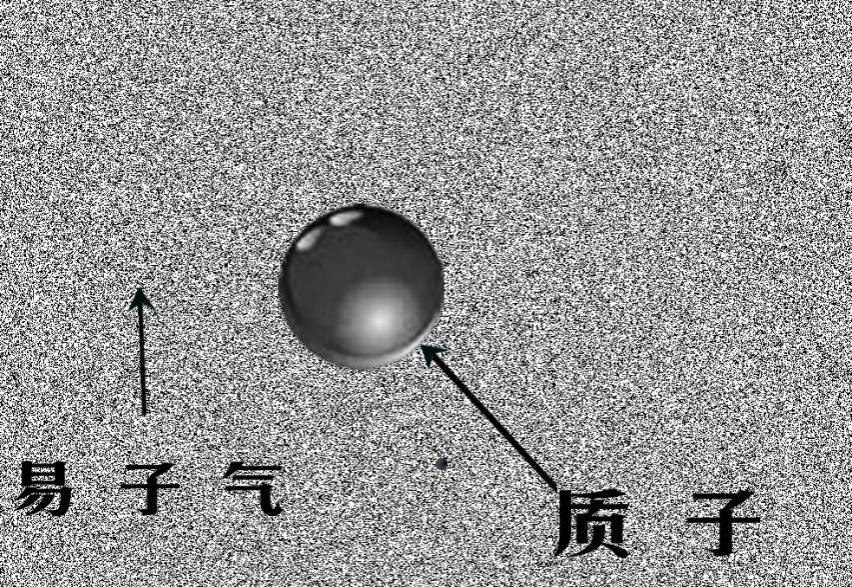Fig.4 Protons suspend in Yizi gas.

3.1. Our universe is a mixture of protons, electrons and Yizi. Most protons and electrons suspend in Yizi gas in different material forms, as shown in figure 4.When a single proton suspends in Yizi gas, since the volume of the proton is much larger than Yizi, a proton collids with a large number of Yizi around it at the same time. The cooperative force of Yizi on the proton tends to be balanced, the kinetic energy of the proton is very small, and the temperature tends to zero (cosmic background temperature). At the same moment, the number of collisions of protons, in a certain line, in opposite two directions is n1 and n2 respectively, and set up n=|n1-n2|, then the momentum of a proton tends to be equal to that of n Yizi:

M proton×v proton ≈ n×mYizi×VYizi —— 1

Because n1 and n2 tend to be the same, so the value of n is small,

And because: Mproton ≫ n mYizi

So: vproton ≪VYizi ——2

From 1×2, we get:

Mproton × vproton × vproton ≪ n×mYiziVYizi × VYizi

That is,×Mproton × vproton2 ≪× n × mYizi VYizi2 ——3

At this time, the energy of n Yizi is much larger than the energy of a proton.That is to say, when a single proton suspends in Yizi gas, the momentum of the proton tends to be equal to that of n Yizi, while the kinetic energy of the proton is much less than that of n Yizi, and the temperature of the proton tends to absolute zero, although the energies of Yizi around the proton are very high.

3.2. And when the multiple protons are completely separated from the Yizi gas, since there is Yizi gas on one side of the proton and no Yizi gas on the other,that is to say n1=0，n2=n.The proton’s one side collides with n2 Yizi at the same time, and the kinetic energy of n2 Yizi is much greater than that of the individual proton suspends in the gas. As shown in Eq.3, so the proton will continuously absorb energy from the YiZi gas, so that the kinetic energy of a proton tends to be equal to the kinetic energy of n2 YIzi, and the pressure tends to be equal, as shown in figure 5:

Now×Mproton×vproton≈× n×mYizi VYizi 2 -------④

Because  vproton ≪VYizi--------⑤

So ④÷⑤ get：M proton×vproton ≫nmYizi×VYizi- --------⑤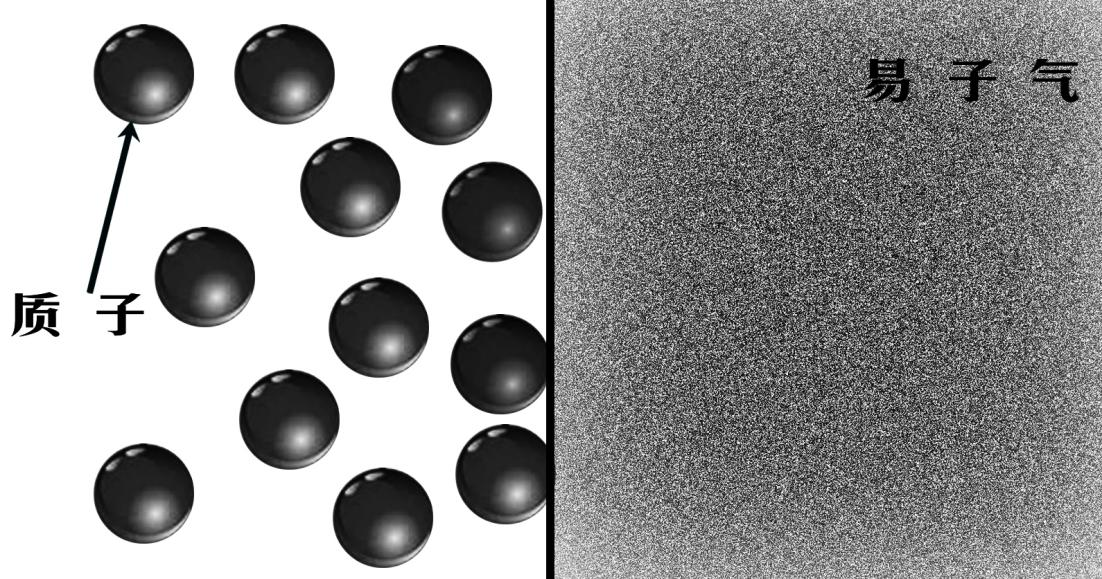Fig.5 Proton gas and Yizi gas are distributed separately.

That is to say, when multiple protons and commuton gas are completely separated, the kinetic energy of a single proton tends to be equal to that of n commutons, while the momentum of a single proton is far greater than that of n commutons.

The greater the mass of the object, the less the Yizi gas that penetrates into the interior of the object, and the greater the difference in the Yizi density of the inner and outer parts of the object. More Yizis transfer kinetic energy to the proton, and the temperature of the object is higher. The object absorbs energy in the form of a direct collision and then releases the energy in the form of thermal radiation to form a dynamic equilibrium.

4. Three physical experiments prove the existence of Yizi

4.1. Experiment 1: The gravitational interaction between two objects is not only related to the masses of the two objects but also to the ambient temperature at which the two objects are located. The force between the two objects is mutually exclusive when their temperature is lower than the ambient temperature.

4.1.1. Experimental equipment and environment: The vacuum tank as shown in Fig.6. the inner diameter is 1.8m and the inner height is 1.8m. It is placed in a constant temperature cave. The gravitational torsion scale (Cavendish torsion scale) is placed on a small table in the vacuum tank as shown in Fig.7. The light is injected from the outside of the vacuum tank onto the small mirror on the torsion scale, and reflected to the opposite side on the whiteboard.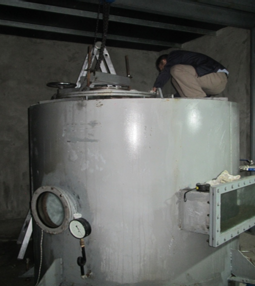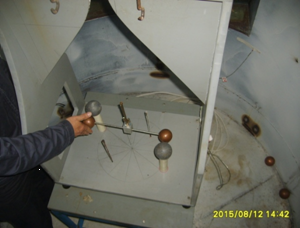Fig.6 Vacuum tank with a torsion scale placed in.           Fig.7 A homemade Cavendish torsion scale placed in the vacuum tank.

4.1.2. Basic parameters and principles of the experiment:

As shown in Fig.8 and Fig.9: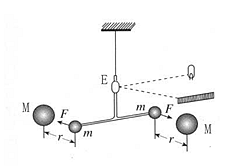Fig.8 working principle diagram of Cavendish torsion balance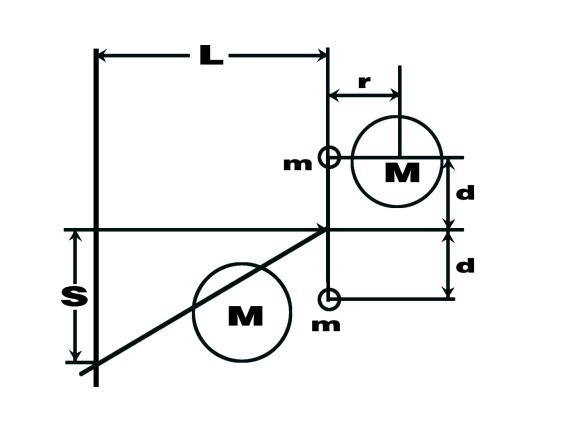Fig.9 vertical dimension of the torsion balance

4.1.2.1. The size of gravity F,

4.1.2.2. The distance of the reflective plane mirror from the whiteboard L=10.3m

4.1.2.3. The small copper ball mass m=0.575kg, the large lead ball mass M=1.5kg

4.1.2.4. Torsion balance arm length d = 0.15m

4.1.2.5. The free vibration period of the torsion balance is T=214s

4.1.2.6. S is the amount of movement of the scale light spot

4.1.2.7. The distance between the center of mass of the big ball and the small ball is r.

4.1.3. Matters need attention:

4.1.3.1. When the gravitation torsion scale is placed in a vacuum tank with constant temperature, it does not change the ambient temperature or the temperature of the metal ball, the big metal ball and the small metal ball attract each other, because the temperature of the metal ball is slightly higher than the temperature of the surrounding Yizi gas.The larger the mass of an object in a constant vacuum, the higher its central temperature will be. This will be proved in the following experiment.

4.1.3.2. The vacuum degree of the vacuum tank should be less than 1000Pa. If there is too much residual air, when the ambient temperature changes, the air around the large metal ball and the small metal ball will turn over, affecting the experimental results.

4.1.3.3. There should be no objects around the metal sphere other than the one used to measure gravity, otherwise the experiment will be complicated and even lead to the opposite result.

4.1.3.4. Not all metals are suitable for gravitational experiments. For example, metal aluminum has a repulsive effect on metal lead itself, so neutral metals are more suitable for gravitation experiments.

4.1.3.5. Slowly inject ice water or hot water to avoid the obvious Thomson effect and increase the additional gravity, which will have a great impact on the experiment.

4.1.4. Experimental steps:

4.1.4.1. Remove the two large lead balls from the torsion scale, leaving only two small copper balls on the T-frame of the torsion scale.

4.1.4.2. Vacuum the vacuum tank with a vacuum degree below 1000Pa.

4.1.4.3. It will take 72 hours for the torsion scale to stop swinging in the vacuum. When the torsion scale stops swinging, mark the position of the light spot on the white board and draw a vertical line in red to represent the balance position.

4.1.4.4. Send hot air to the insulating room to do basic test on the whole experimental system. There is no obvious change in the point of light, so the influence of the system on it can be ignored.

4.1.4.5. Turn off the air conditioner, open the vacuum tank, put the big ball on the torsion scale, the center distance between the big ball and the small ball is about 10CM, then close the vacuum tank and vacuum it.

4.1.4.6. After standing in the natural environment without heating or cooling for 72 hours, it was found that the light spot shifted to the direction of attraction by about 0.4-0.5cm, S≈0.0045m.

Gravitation force in the natural state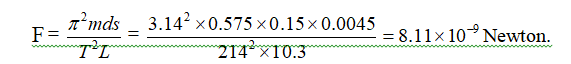4.1.4.7 At this time, sent hot air to the insulating room. The temperature inside the room rises by 3 to 4 degrees in 3 minutes, and the temperature inside the vacuum tank rises less than 0.1 degree. At this time, the temperatures of the big ball and the small ball are lower than the environment in which they are located. The light spot move 3.5CM in the direction in which the big ball and the small ball repel each other. As the temperature in the room increases, the maximum moving distance of the light spot to the repulsive direction is about 15CM, as shown in Fig.10.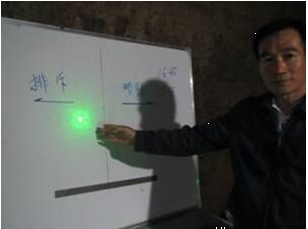Fig.10 When external temperature of the vacuum tank rised,the light spot moves in repulsive direction

The repulsive force F which generated by the light spot moving 15CM: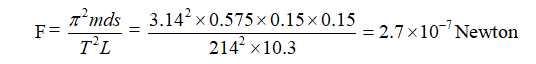This repulsive force is 33 times the gravitational force measured at the natural ambient temperature.

4.1.4.8. Turn off the air conditioner and let the heat insulating room stand still.

After two days, send cold air to the insulating room. As the temperature of the room decreases, the gravity between the big ball and the small ball increases, and the light spot moves in the direction of mutual attraction, and the maximum moving distance reaches 17CM. This attractive force is 37 times of the gravity measured in the natural environment temperature.

4.2. Experiment 2: Change the temperature of the big metal ball on the gravitation torsion scale, the temperature of the small metal ball is unchanged, observe the effect of the big metal ball on the force of the small metal ball (when the temperature of the big metal ball increases, it will attract the small ball; when the temperature of the big metal ball decreases, it will repel the small ball).

4.2.1. Laboratory equipment and environment: A constant temperature room built in a cave. A vacuum tank, placed inside the constant temperature room, with a gravitational torsion scale placed inside it. A hanging wire hang a T-frame on the torsion scale, and at the two ends of the T-frame, there is a small copper ball respectively, as shown in Fig.11. One of the small copper balls has a large hollow copper ball on its side. The large copper ball has an inlet pipe and an outlet pipe, which are used to change the temperature of the large copper ball by injecting hot water or ice water into it through a miniature water pump, as shown in Fig. 12.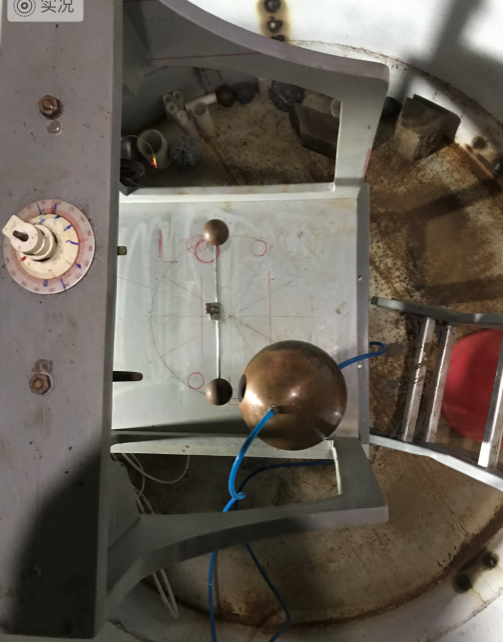Fig.11 Top view of gravity balance in vacuum tank               Fig.12 A small pump and a bucket used to fill in the large hollow copper ball with hot or icy water.

4.2.2 Matters needing attention

4.2.2.1The vacuum degree of the vacuum tank should be less than 1000Pa. If there is too much residual air, when the ambient temperature changes, the air around the large metal ball and the small metal ball will turn over, affecting the experimental results.

4.2.2..2 There should be no objects around the metal sphere other than the one used to measure gravity, which would complicate or even reverse the experiment.

4.2.2.3 The large hollow copper ball must be connected with the ground wire to avoid electron transfer and electrostatic attraction inside the hollow copper ball caused by injection of ice water or hot water.

4.2.3. Experimental steps:

4.2.3.1. The center of the large hollow copper ball placed on the torsion balance is on the same horizontal plane as the center of the small copper ball on the t-frame, as shown in Fig.13.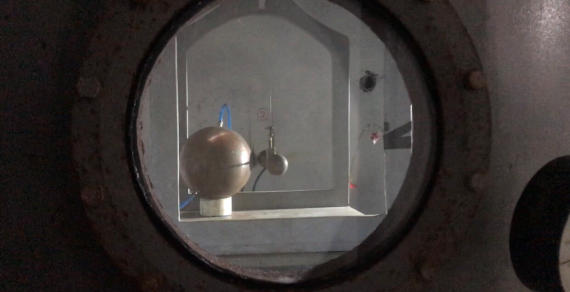Fig.13 The center of the large hollow copper ball is on the same level as the center of the small copper ball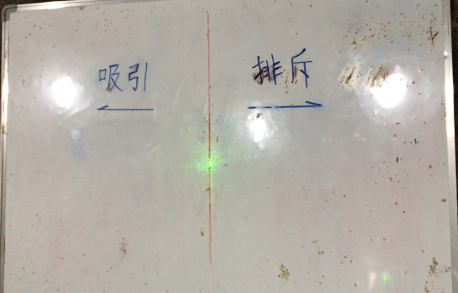Fig.14 The small copper ball below the suspension stops swinging and the light spot stays on the midline.

4.2.3.2. After being placed for a long time, the small copper ball under the suspension wire has stopped swinging, and the light spot stays on the middle line of the white board, as shown in Fig.14.

4.2.3.3. When we inject hot water into the large hollow copper ball, it attracts the small copper ball, and the light spot moves in the direction of attraction.

4.2.3.4. When we inject ice water into the large hollow copper ball, it repels the small copper ball, and the light spot moves in the direction of repelling.

4.3. Experiment 3: Under the condition of constant temperature and vacuum, the temperature of an object is related to its mass. The larger the mass of an object, the higher its temperature will be.

4.3.1. Laboratory equipment and environment: a homothermal room built in the cave, a vacuum tank placed in the homothermal room; a vacuum pump, a temperature collector and computer are placed outside the room; a 1000kg copper ball and a 1.2kg copper ball, both are placed in the vacuum tank as shown in Fig.15.

4.3.2. Matters need attention: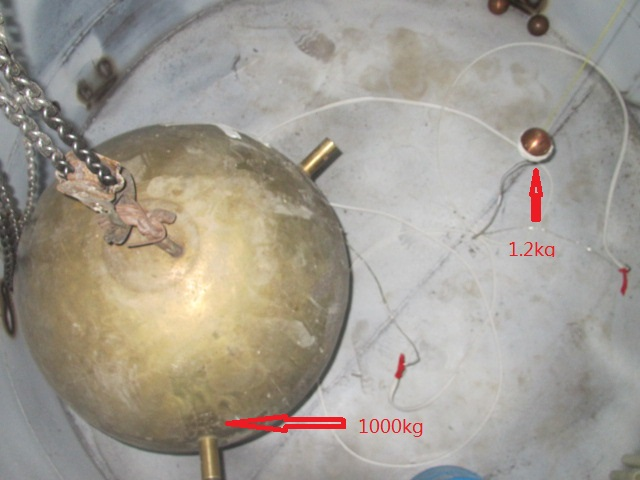Fig.15 A 1000kg copper ball and a 1.2kg copper ball are suspended in a vacuum tank.

4.3.2.1. Thermistor  is very sensitive, and its temperature measuring error is 0.002degree, so all electric lines must be welded, the main circuit must use electrostatic shielding wire, and the joints shall be insulated.

4.3.2.2. Copper balls are used in this experiment mainly because of the high thermal conductivity of copper. The temperature reaches the equilibrium state quickly and its price is moderate. There are three main ways of heat transfer: heat conduction, convection, and heat radiation. Here, in order to maintain a constant temperature, we place the large and the small copper ball in a vacuum tank, suspend them, and let them balance the temperature mainly in the form of heat radiation. The center of the large and the small copper balls should be on the same horizontal plane, which mainly takes into account the inconsistency of the ambient temperature at different horizontal planes.

4.3.2.3. The current flowing through the thermistor should be as small as possible,otherwise, the current will generate the same amount of heat. The small copper ball will be warmer than the large copper ball, because it can not dissipate as much as the large ball. Here the thermistor carries a current of 0.0104 mA.

4.3.2.4. The 24-hour temperature change of the vacuum tank in the homothermal room shall not exceed 0.2 degrees.

4.3.3. Experimental steps:

4.3.3.1. Place the large copper ball and the small copper ball in the vacuum tank and keep their centers at the same level. The thermistor R1 attaches to surface of the large copper ball, and the thermistor R2 attaches to surface of the small copper ball.

4.3.3.2. Close the vacuum tank and evacuate it to a vacuum of -0.1 MPa.

4.3.3.3. Because the copper balls have been placed in the cave for a long time, the heat balance will not take too much time, and 24 hours is enough. After 24 hours, the temperature curve shown in Fig.16 is recorded by the thermistor collector and computer. The red line indicates the temperature change curve of the large copper ball with time. The blue line is the temperature change curve of the small copper ball with time. The average temperature of the large copper ball is about T1=10.13, and the average temperature of the small copper ball is about t1=10.04.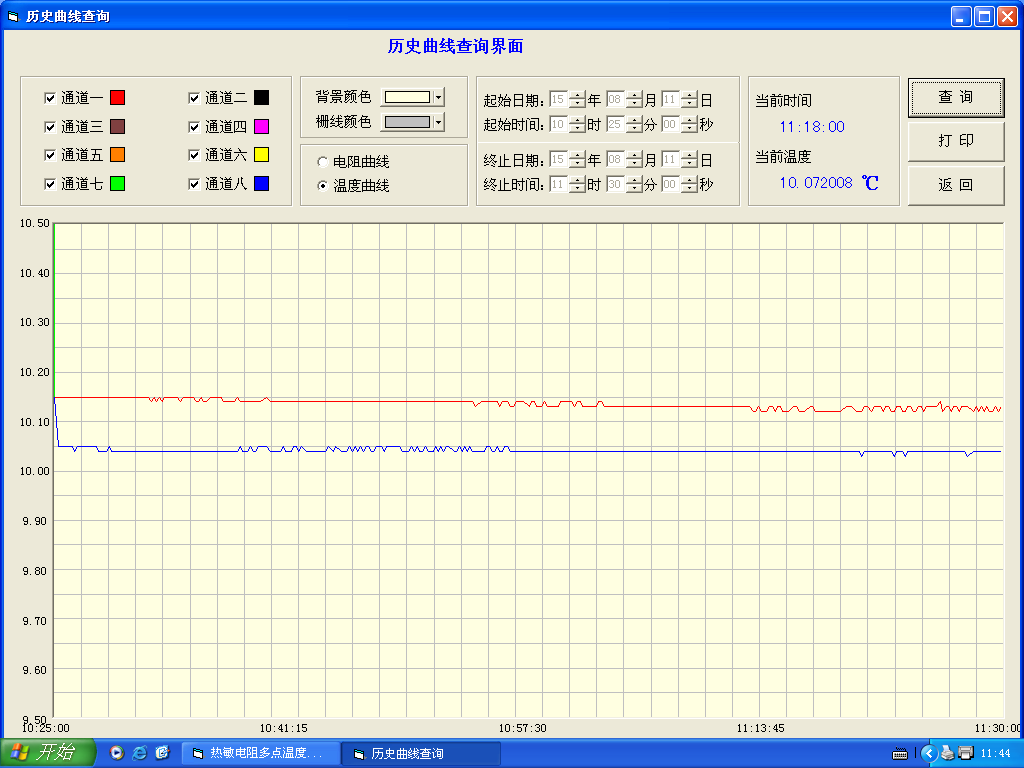Fig.16 The temperature curve of the big copper ball and the small copper ball over time. The red line represents the temperature of the big copper ball and the blue line represents the small copper ball.

4.3.3.4. Open the vacuum tank, reverse the two resistances. Attach the resistor R1 to the surface of the small copper ball, and measure its temperature. Attach the resistor R2 to the surface of the large copper ball, and measure its temperature. Close the vacuum tank, vacuum, and check the temperature record after 24 hours.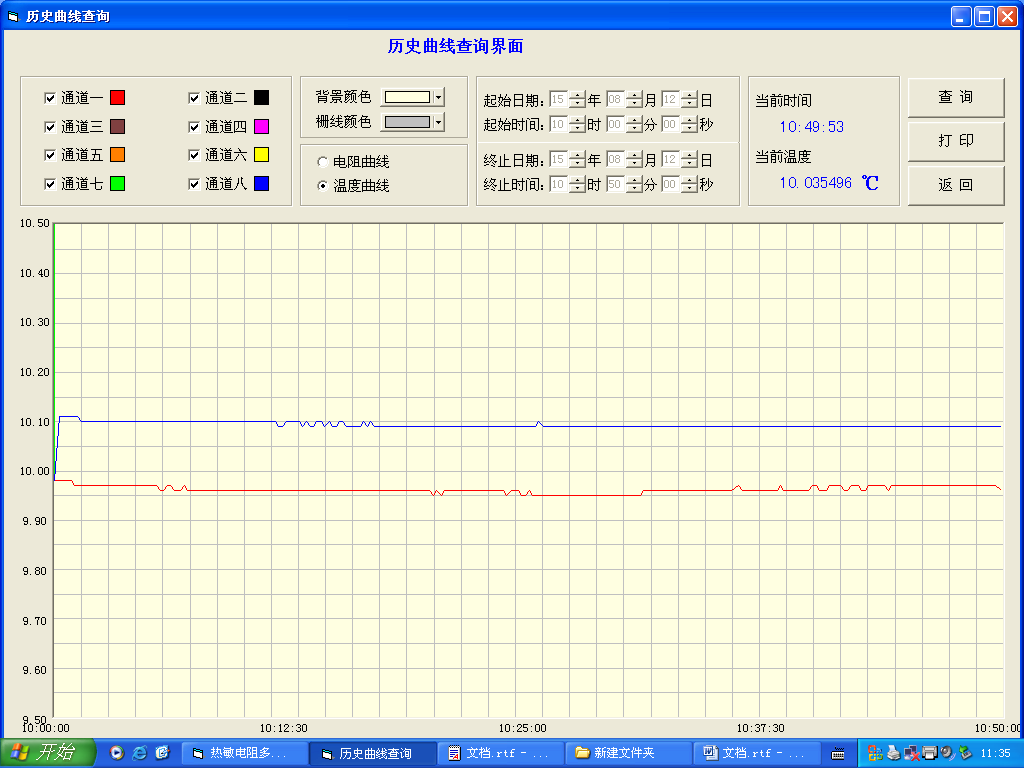Fig.17 The temperature curve of the big copper ball and the small copper ball over time. The blue line represents the temperature of the big copper ball and the red line represents the small copper ball.

4.3.3.5. After 24 hours, query the computer, as shown in Fig.17; the blue curve shows the temperature curve of the large copper ball change with time. The average temperature of the large copper ball is about T2=10.07. The red line is the temperature curve of the small copper ball change with time, and its average temperature is about t2 = 9.97. The average temperature difference between the two balls measured twice.

The temperature of the large copper ball of 1000 kg is 0.095 degrees higher than the small copper ball of 1.2 kg.

The two thermistors are swapped for the purpose of eliminating system errors because the temperatures measured by the two thermistors are erroneous at the same temperature.

The above experiments proved that under constant temperature and vacuum conditions, the greater the mass of the object, the higher the temperature of the object, and the greater the gravitational force formed. Gravity can only exert force within a certain distance. When an object is too far away from the planet, the force on the object will become zero when the microwave in the space where the object is located is the same as the microwave intensity generated by the Yizi gas at the cosmic background temperature.

5. The physical properties of Yizi

5.1. The trajectory of Yizi movement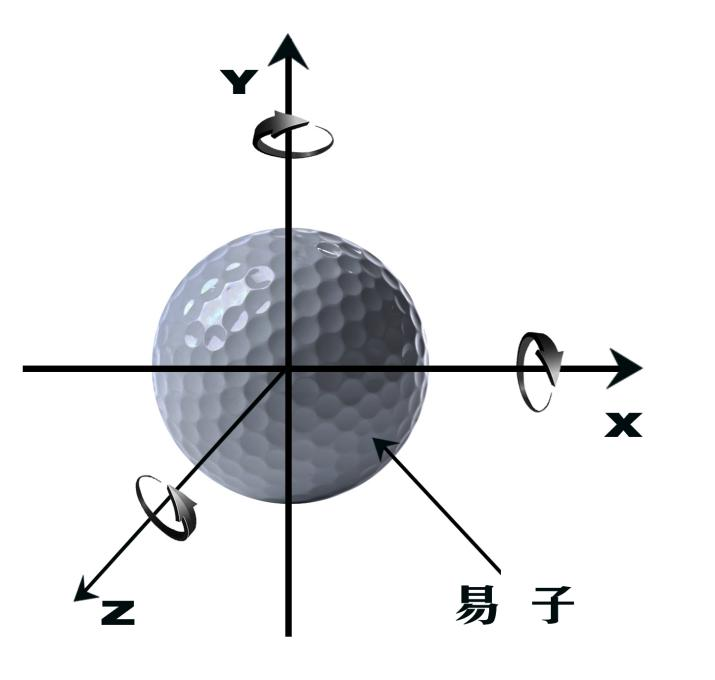Fig.18 Yizi model

Assume that the Yizi is a rough surface sphere, as shown in Fig.18. In addition to translation, the movement of the Yizi also has an eccentric rotation motion of the Yizi around the x-axis, the y-axis, and the z-axis. The eccentric rotation makes the movement trajectory of the Yizi in each plane to be a circle. The circles in three planes combin into a closed ellipse, so the trajectory of the Yizi is a closed ellipse, instead of a straight line. The Yizi has not only a high translational speed, but also a higher edge velocity when rotating.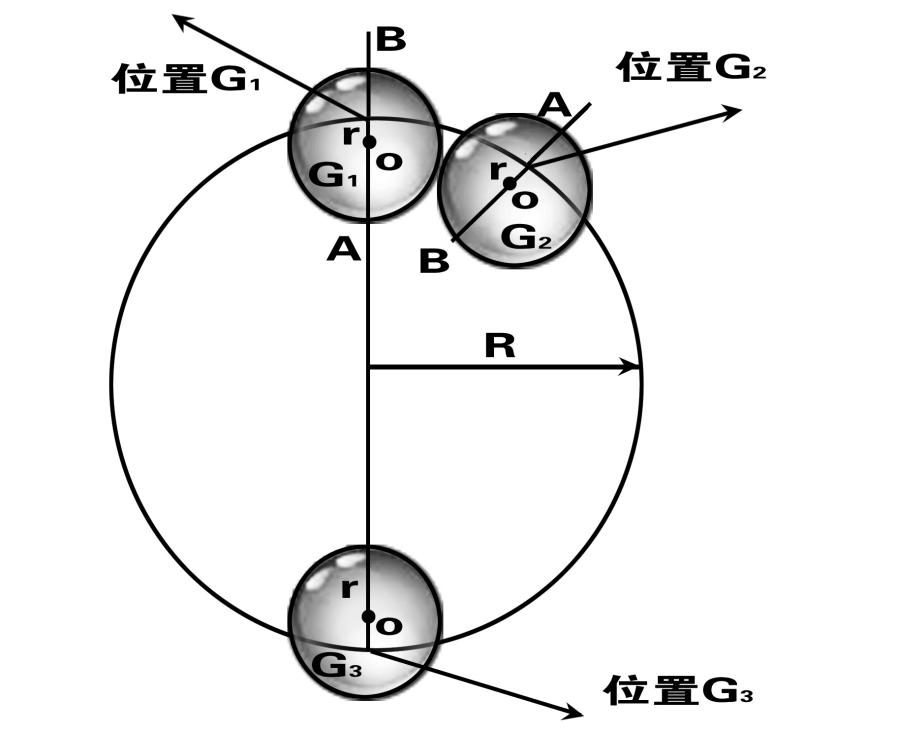Fig.19 Plane movement trajectory of Yizi.

When Yizi collide with each other, they transfer not only translational momentum,but also angular momentum. The edge collision of Yizi makes it have a very high rotational speed. As shown in Fig.19, suppose that Yizi moves in a circle. When Yizi is in position G1, the angular momentum of A is greater than that of B.The commutator rotates in half a circle and the angular momentum is transferred once, the angular momentum at A is transferred to B, and B transfers angular momentum to A.When Yizi moves to the position G2, it rotates n+1/2 times. At this time, the angular momentum of the A end is converted to B, and the angular momentum of the B end is converted to A. During this conversion between the A end and the B end, the angular momentum of Yizi in the inner side of the large circle R is always greater than the angular momentum outside the large circle. This inner and outer angular momentum difference only changes the direction of motion,  but it has no effect on the magnitude of the inner and outer angular momentum. The eccentricity r and the radius R of the Yizir's circular motion satisfy the following formula,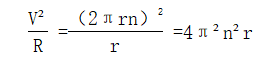where V is the plane motion rate of the Yizi, R is the radius of the circular motion of the Yizi eccentricity point, r is the eccentricity of the Yizi rotation, and n is the number of rotation times of the Yizi revolution.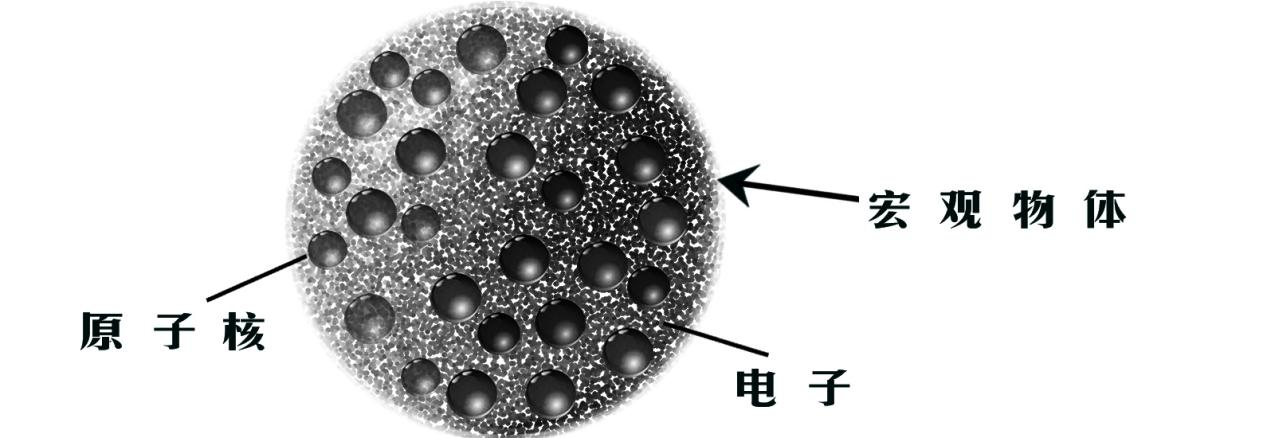Fig.20 A macroscopic object composed of proton electron commutons

The same is true for electrons and protons. If the surface is rough and the trajectory is a closed ellipse, it is not surprising that large angles of scattering occur in collisions. There is no straight linear motion in the micro world. Then why does the macro world have a linear motion? Since the macroscopic objects are composed of many particles,as shown in Fig.20, when the macroscopic object rotates, the particles in the object collide with each other, and the angular momentum in the object is quickly distributed uniformly, so that the object rotates around the centroid.

5.2. Average Speed of Yizi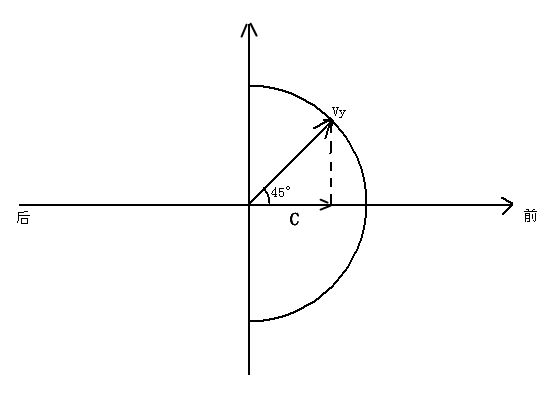Fig.21 Schematic diagram of the relationship between the average velocity of commutons and the speed of light.

Light is transmitted by Yizi gas, just as sound is transmitted by air. The average speed of Yizi's irregular movement in Yizi gas determines the speed of light propagation in Yizi gas. The light will be like a sound, gradually weakening in the spread, and eventually dissipate in Yizi gas. The speed of sound propagation in the air is related to the rate of thermal motion of air molecules. As the temperature increases, the rate of thermal motion of air molecules increases and the speed of sound increases. Light is transmitted by Yizi gas, and a large number of Yizi do irregular movements colliding with each other. Unlike air molecules, air molecules do elastic collisions, while Yizi does hard collisions, and there is no time of relaxation. The opportunity for Yizi to move in all directions is equal. If the direction of movement of Yizi is divided into two directions, the average direction of movement of the Yizi with a tendency to move forward should be 45°angle in the forward direction, as shown in Fig.21, where Vy is the average velocity of YiZi, C is the speed of light, and the average propelled forward velocity C=Vy , is the speed of light. The average speed of Yizi is Vy=c= 4.24 x 108m/sec.

5.3. The mass of Yizi

We know the energy carried by electromagnetic wavesε=hγ, where h is the Planck constant and γ is the frequency of the electromagnetic wave. In fact, h is the minimum average energy that electromagnetic waves can carry, that is, the average kinetic energy carried by per Yizi, so h=myvy2, where my is the mass of the Yizi.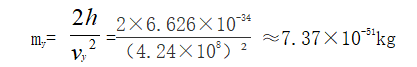References:

 Fundamental particles : Edited by Cen yisheng and Lizengzhi University Physics Edited the second volume of Tianjin university press 1999.2

 The interstellar cloud: Edited by lee chongwei and Xiao xinghua Astrophysics,Beijing higher education press 2000

 Electron cyclotron: Edited by Cen yisheng and Lizengzhi University Physics Edited the second volume of Tianjin university press 1999.2

 Brownian motion: Edited by Cen yisheng and Lizengzhi University Physics Edited the second volume of Tianjin university press 1999.2

 Cosmic background radiation of 2.735k: Edited by lee chongwei and Xiao xinghua Astrophysics,Beijing higher education press 2000

 Planck's constant: Edited by Cen yisheng and Lizengzhi University Physics Edited the second volume of Tianjin university press 1999.2

 the speed at which light travels: Edited by Cen yisheng and Lizengzhi University Physics Edited the second volume of Tianjin university press 1999.2

 grand unified theory: Edited by Zhangli Progress in modern physics,Beijing Tsinghua university press 1997

 thermistors: Edited by Wang cu、Li chun and Zhou lezhu.Electromagnetics.Peking university press 2000.2

ε=hγ: Edited by Jin zhonghui、Liang deyu,University basic physics.Beijing science press,2000.5

 上一页      回目录      下一页
 ------------------------------------------------------------------------------------------------------------------------------------------------
 Copyright (C) 2004 rights reserved.李华旺 版权所有 电话:086-0411-82641525 E-mail:lhw@dlhuaxin.com 易子论网（李华旺） 地址:中国大连中山区明泽街16号 丽苑大厦5楼H座 邮编:116001 Author: li Huawang Address: 5H, Liyuan Mansion, No.16, Mingze Street, Zhongshan District, Dalian City Postcode: 116001 E-mail:lhw@dlhuaxin.com 辽ICP备12005732号